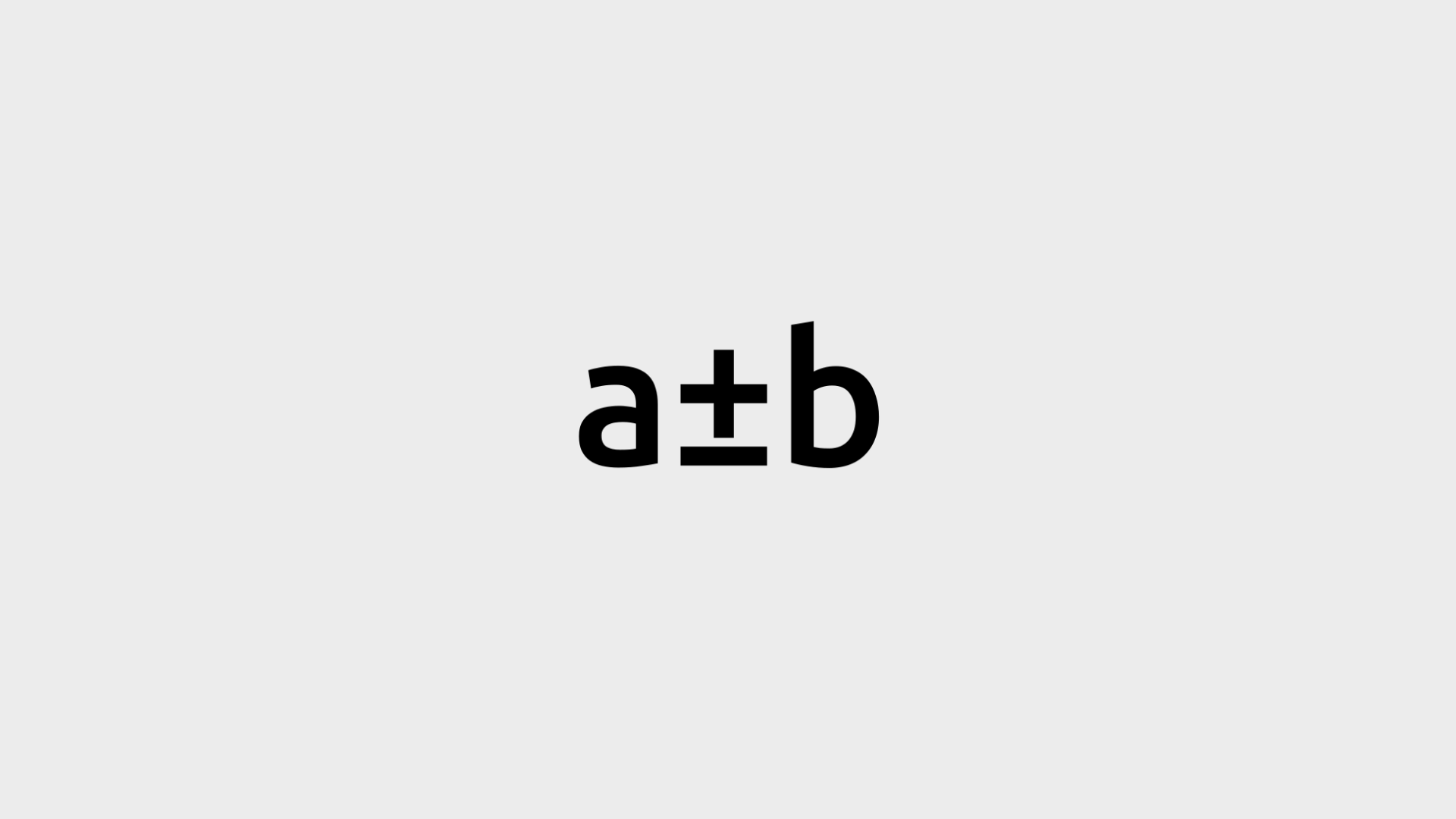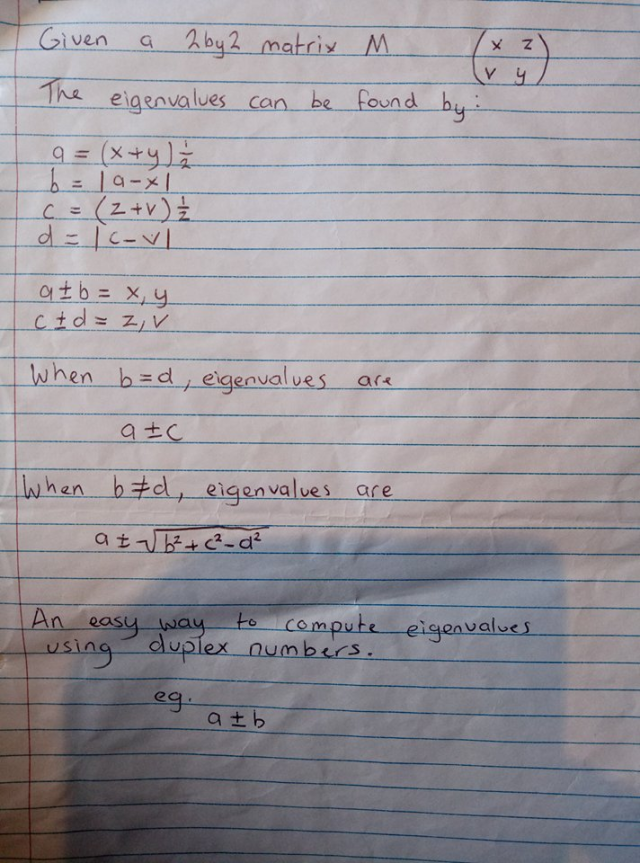A duplex number is a type of number that I invented that can represent many numbers at once. For example, 5±2 represents 7 and 3. Each ‘±’ symbol here, denotes a branch where either operation (plus and minus) can be taken to reach a represented value.

They can be chained together as well. For example, 7.5±4±2±1±0.5 can represent all the numbers from 0 to 15.

Applications
Duplex numbers can be used to calculate the eigenvalues of a 2x2 Matrix. This method is simpler than the traditional method and may hint at different geometric interpretations of what an eigenvalue is..

Attributes
The first part of a duplex number is the mean of the distribution of numbers that it represents. The sum of the squares of any subsequent parts of the duplex number is equivalent to the statistical variation of the distribution. Duplex numbers can be scaled and combined with standard numbers.

It makes some sense to multiply unchained duplex numbers but a standard multiplication framework has not been identified for the numbers yet.

Thoughts
I think the numbers may have some relationship with the Qubits found in Quantum Computing. Both Qubits and Duplex Numbers are capable of representing many different values at the same time.

There is a lot of potential for them. I hope the numbers will have further applications and uses. I don’t have the mathematical background to spot where else they may fit in the field.